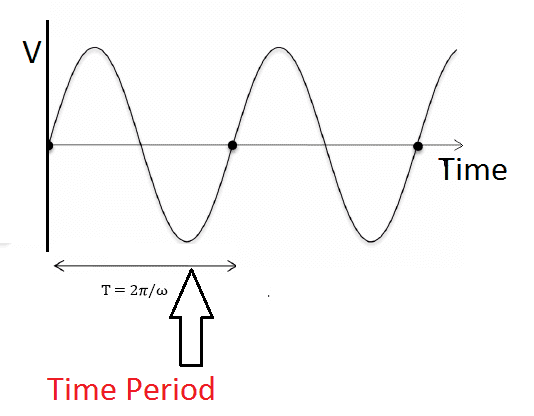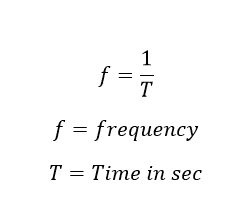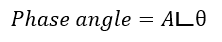# Alternating current’s important terms and condition’s definition:

Alternating current is nothing but the current flows through the conductor changes its direction periodically. In alternating current, the electrons don’t move in only one direction. Instead, they hop from atom to atom in one direction for a while, and then turn around and hop from atom to atom in the opposite direction. Every so often, the electrons change direction. In alternating current, the electrons don’t move steadily forward. Instead, they just move back and forth. To study about alternating current we must know about some important terms.

1) Waveform

2) Instantaneous value

3) Cycle

4) Time period

5) Frequency

6) peak value

7) Peak to peak value

8) RMS value

9) Peak Factor

10) Form factor

11) Phase

12) Phase Difference

13) In Phase

14) Out of phase

## Waveform:

A plot or graph or shape and form of signal is obtained by plotting the instantaneous values of the quantity such as current and voltage with the frequency, against a base of time or angle is called Waveform. A waveform is the shape and form of a signal such as a wave moving in a physical medium or an abstract representation.

Learn More:   7 Purpose of NGR Neutral Grounding Resistor Transformer & Generator

Read the main article: Wave Form

## Instantaneous value:

The magnitude of the waveform has taken at any point of time is called instantaneous value. And the instantaneous value of the alternating quantity is designated by a lower case letter small e for emf, small v for voltage and small i for current.

Read the main article: Instantaneous value

## Cycle:

When a waveform reaches a complete set of positive and negative value it is called one cycle., such as if a sinusoidal wave, completes one set of positive and negative values, is called one cycle of sine wave cycle. One cycle is corresponding to the angular measure of 360 deg.

## Time Period:

The time taken to complete one cycle of an alternating quantity is called time period. The time period is denoted by T.## Frequency:

The number of cycles completed per second by an alternating quantity is known as frequency and is denoted by f. In SI system, the frequency is expressed in Hz that is the short form of hertz (pronounced as hurts). The wave’s frequency is said to be One hertz (or Hz), which is equal to one cycle per second. The number of cycles completed per secondAlso, the reciprocal of the time period can be called as frequency.

The general utility of AC power supply system is produced at a frequency of 50 Hz or 60 Hz. But in the world, the major country uses 50 Hz power system, In US, brazil, Canada such countries use 60 Hz power system.

Also see: 50 Hz power system vs 60 Hz power system. Why India has 50 Hz power system.

The output of the equipment increases with the increase in frequency. The output voltage is directly proportional to the frequency of the machine. For a given output, smaller size machines are required as compared to those for lower frequency output. Because of high power-weight ratio, the relative cost of the equipment is also reduced.

At the same time increase in frequency, the loss of the electrical circuit also increases.

Lower regulation, lower skin effect resulting in lower ohmic losses (resistive losses), lower magnetic and dielectric losses resulting in higher efficiency, lower corona loss and higher power transmission line capacity as compared to those at higher frequency.

## Peak Value:

The maximum value of the sin wave’s positive cycle or negative cycle.

## Peak Value: amplitude measurement

The maximum value, which is measured from positive peak to negative peak value of the waveform. Peak-to-peak value is the measure between peak (highest amplitude value) and lowest amplitude value, which can be negative. With appropriate circuitry, peak-to-peak amplitudes of electric oscillations can be measured by meters or by viewing the waveform on an oscilloscope.

Peak-to-peak is a straightforward measurement on an oscilloscope, the peaks of the waveform being easily identified and measured in Oscilloscope. This remains a common way of specifying amplitude, but sometimes other measures of amplitude are more appropriate.

## What is Phase:

The phase is defined as the position of the waveform at a fraction of time period. Phase is expressed in angle or radian. Phase can also be an expression of relative displacement between two corresponding features (for example, peaks or zero crossings) of two waveforms having the same frequency.

## Phase difference:

The phase difference is the difference, between two waves is having the same frequency and referenced to the same point in time. It is expressed in degrees or radians

## Phase angle:

In the context of phasors, phase angle refers to the angular component of the complex number representation of the function. The notation of the phase angle is defined as## In phase:

The two waveform said to be in the phase where the two wave should reach maximum, minimum and zero values simultaneously at the same time.

## Out Phase:

Two sinusoidal signals are said to be out of phase when they do not reach maximum or zero values at the same time.